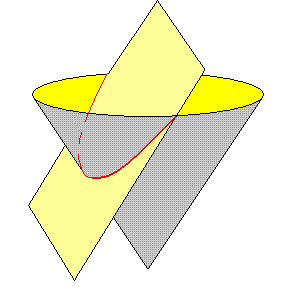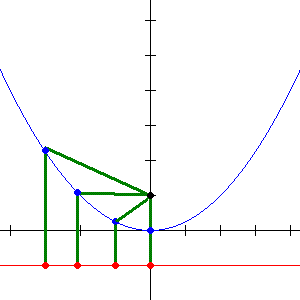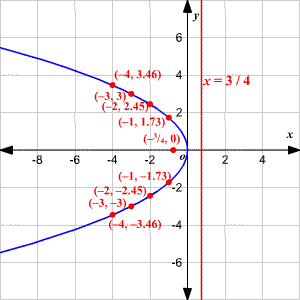# Parabolas as Conic Sections

A parabola is the curve formed by the intersection of a plane and a cone, when the plane is at the same slant as the side of the cone.A parabola can also be defined as the set of all points in a plane which are an equal distance away from a given point (called the focus of the parabola) and a given line (called the directrix of the parabola).In beginning algebra, we usually consider only parabolas whose axis of symmetry is vertical. The equations for these curves are in the general form

$y=a{x}^{2}+bx+c$ ,

where $a,b$ and $c$ are constants.

The standard form of the equation of a parabola with a vertex at $\left(0,0\right)$ is as follows.

 Equation Focus Directrix ${x}^{2}=4py$ $\left(0,p\right)$ $y=-p$ Vertical axis ( $x=0$ ) ${y}^{2}=4px$ $\left(p,0\right)$ $x=-p$ Horizontal axis ( $y=0$ )

Example 1:

Find the focus and directrix of the parabola $y=-2{x}^{2}$ .

Since the $x$ -term is squared, the axis is vertical, and the standard form is

${x}^{2}=4py$

Rewriting the equation in standard form:

${x}^{2}=-\frac{1}{2}y$

Comparing the equation with the standard form:

$\begin{array}{l}4p=-\frac{1}{2}\\ p=-\frac{1}{8}\end{array}$

The focus of the parabola which is in standard form ${x}^{2}=4py$ , is $\left(0,p\right)$ .

So, the focus of the equation is $\left(0,-\frac{1}{8}\right)$ .

The directrix of the parabola which is in standard form ${x}^{2}=4py$ , is $y=-p$ .

So, the directrix of the equation is $y=\frac{1}{8}$ .

Example 2:

Graph the equation and then find the focus and directrix of the parabola ${y}^{2}=-3x$ .

The equation is of the form ${y}^{2}=4px$ where $4p=-3$ .

Solving for $p$ :

$p=-\frac{3}{4}$

The focus of the parabola which is in standard form ${y}^{2}=4px$ , is $\left(p,0\right)$ .

So, the focus of the equation is $\left(-\frac{3}{4},0\right)$ .

The directrix of the parabola which is in standard form ${y}^{2}=4px$ , is $x=-p$ .

So, the directrix of the equation is $x=\frac{3}{4}$ .

Since the variable $y$ is squared, the axis of symmetry is horizontal. Also the value of $p$ is less than $0$ , the parabola opens to the left.

Choose negative $x$ -values and make a table.

 $X$ $-1$ $-2$ $-3$ $-4$ $Y$ $1.73$ $2.45$ $3$ $3.46$

Plot the points and draw a parabola through the points.